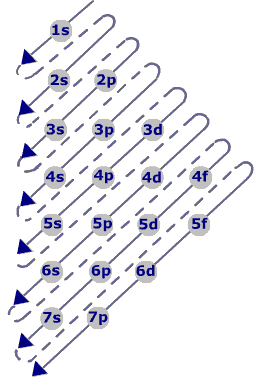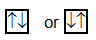Click to Chat

1800-1023-196

+91-120-4616500

CART 0

• 0

MY CART (5)

Use Coupon: CART20 and get 20% off on all online Study Material

ITEM
DETAILS
MRP
DISCOUNT
FINAL PRICE
Total Price: Rs.

There are no items in this cart.
Continue Shopping```Electronic Configuration

Table of Content

Rules for filling of electrons in various orbitals

Aufbau Principa

Pauli’s Exclusion Principle

Hund’s Rule of Maximum Multiplicity

Exceptional Configurations

Rules for filling of electrons in various orbitals

Refer to the Following Video for electronic configuration

Aufbau PrincipaThis principle states that the electrons are added one by one to the various orbitals in order of their increasing energy starting with the orbital of lowest energy. The increasing order of energy of various orbital is 1s, 2s, 2p, 3s, 3p, 4s, 3d, 4p, 5s, 4d, 5p, 6s, 4f, 5d, 6p, 5f, 6d, 7p……………………

How to remember such a big sequence? To make it simple we are giving you the method to write the increasing order of the orbitals. Starting from the top, the direction of the arrow gives the order of filling of orbitals.Alternatively, the order of increasing energies of the various orbitals can be calculated on the basis of (n + l ) rule.

The energy of an orbital depends upon the sum of values of the principal quantum number (n) and the azimuthal quantum number ( l ). This is called (n +l ) rule. According to this rule,

“In neutral isolated atom, the lower the value of (n + l ) for an orbital, lower is its energy. However, if the two different types of orbitals have the same value of (n +l ), the orbitals with lower value of n has lower energy’’.

Type of orbitals

Value of n

Values of l

Values of (n+l)

Relative energy

1s

1

0

1 + 0 = 1

Lowest energy

2s

2

0

2 + 0 = 2

Higher energy than 1s orbital

2p

2

1

2 + 1 = 3

2p orbital (n=2) have lower energy than 3s orbital (n=3)

3s

3

1

3 + 1 = 4

Pauli’s Exclusion Principle

According to this principle, no two electrons in an atom can have same set of four quantum numbers. In other words, an orbital can contain a maximum number of two electrons and these two electrons must be of opposite spin. Two electrons in an orbital can be represented byHund’s Rule of Maximum Multiplicity

This rule deals with the filling of electrons in the equal energy (degenerate) orbitals of the same sub shell (p, d and f). According to this rule,
“Electron pairing in p, d and f orbitals cannot occur until each orbital of a given sub shell contains one electron each or is singly occupied”.

This is due to the fact that electrons being identical in charge, repel each other when present in the same orbital. This repulsion can, however, be minimized if two electrons move as far apart as possible by occupying different degenerate orbitals. All the electrons in a degenerate set of orbitals will have same spin.

Electronic Configuration of Elements: Electronic configuration is the distribution of electrons into different shells, subshells and orbitals of an atom.
Keeping in view the above mentioned rules, electronic configuration of any orbital can be simply represented by the notation]Alternatively:

Orbital can be represented by a box and an electron with its direction of spin by arrow. To write the electronic configuration, just we need to know (i) the atomic number (ii) the order in which orbitals are to be filled (iii) maximum number of electrons in a shell, sub–shell or orbital.

Each orbital can accommodate two electrons

The number of electrons to be accommodated in a subshell is 2 x numbers of degenerate orbitals.

Subshell
Maximum number of electrons

s
2

p
6

d
10

f
14

The maximum number of electrons in each shell (K, L, M, N…) is given by 2n2where n is the principal quantum number.

The maximum number of orbitals in a shell is given by n2 where n is the principal quantum number.

The chemical properties of an element are dependent on the relative arrangement of its electrons.

Exceptional Configurations

Stability of half filled and completely filled orbitals

Cu  has  29 electrons. Its expected electronic configuration is

1s2, 2s2, 2p6, 3s2, 3p6, 4s2, 3d9

But a shift of one electron from lower energy  4s orbital to higher energy 3d orbital will make the distribution of electron symmetrical and hence will impart more stability.

Thus the electronic configuration of Cu is

1s2, 2s2, 2p6, 3s2, 3p6, 4s1, 3d10

Fully filled and half filled orbitals are more stable

To read more, Buy study materials of Structure of Atom comprising study notes, revision notes, video lectures, previous year solved questions etc. Also browse for more study materials on Chemistry here
```### Course Features

• 731 Video Lectures
• Revision Notes
• Previous Year Papers
• Mind Map
• Study Planner
• NCERT Solutions
• Discussion Forum
• Test paper with Video Solution